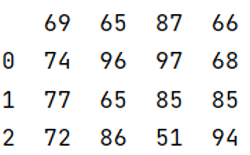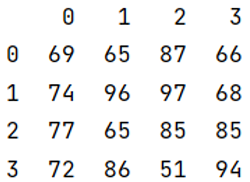Submitted by Pranit Sharma, on May 09, 2022

Pandas is a special tool that allows us to perform complex manipulations of data effectively and efficiently. Inside pandas, we mostly deal with a dataset in the form of DataFrame. DataFrames are 2-dimensional data structures in pandas. DataFrames consist of rows, columns, and the data. DataFrame can be created with the help of python dictionaries or lists but in the real world, CSV files are imported and then converted into DataFrames.

In a CSV file, headers are the values assigned to each column, it is an array of values and it acts as a row or headers. While reading a CSV file, we sometimes don't want the headers, in that case, we do not open the CSV file along with headers, below is the example explaining the implementation.

To work with pandas, we need to import pandas package first, below is the syntax:

```import pandas as pd
```

Let us understand with the help of an example:

```# Importing pandas package
import pandas as pd

# Importing dataset

# Print the dataset
print(data)
```

Output:Now we will pass header = None as a parameter.

```# Importing pandas package
import pandas as pd

# Importing dataset

# Print the dataset
print(data)
```

Output:Preparation